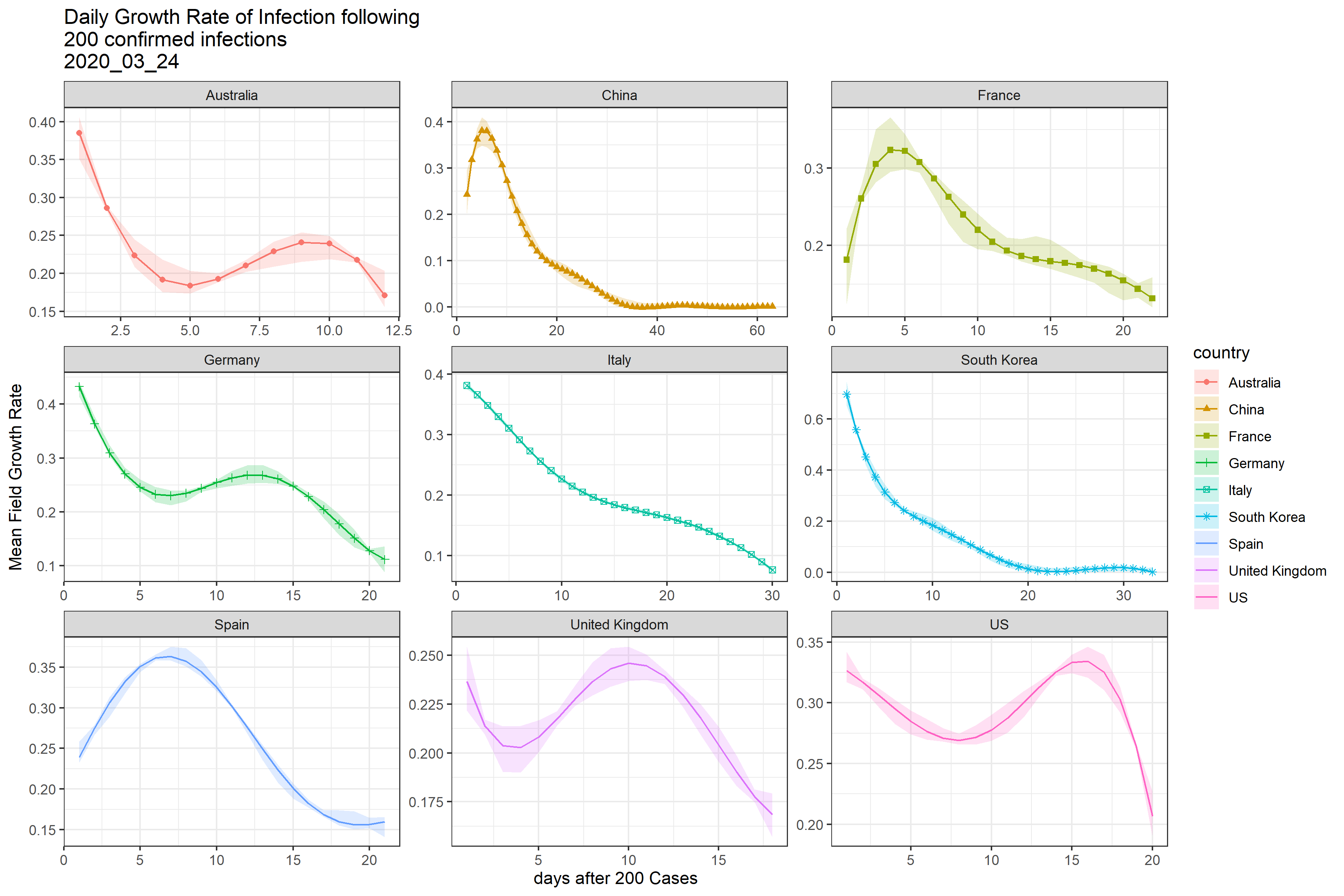# Covid-19 Progression Analysis

Covid-19 Progression Analysis

# Introduction

This updates a prior post analyzing the growth rate of Covid-19 infections and deaths by country.

The analysis is conducted as of 2020-03-24

The daily growth rate of cumulative confirmed cases and deaths are calculated as:

• \(G_{confirmed}=growth~rate~confirmed=log(Confirmed_t / Confirmed_{t-1})\)
• \(G_{deaths}=growth~rate~deaths=log(Deaths_t / Deaths_{t-1})\)

Using this formulation, the total growth after \(d\) days is \(total_{confirmed}=exp(d . G_{confirmed})\) and similarly for deaths. From this formula, we can determine that the number of days to double deaths is \(d^{double}_{deaths} = log(2)/G_{deaths}\). The final plot shows the doubling day period for cumulative deaths.

The analysis uses a Gaussian Process to smooth the growth rates. 95% Credible Intervals for the mean growth rate are also shown.

# Summary of Results

All countries in the analysis except China and South Korea are on a disastrous trajectory. There is some good news from the Italian data which shows a slow but steady improvement in the growth rates of infections and deaths, suggesting the Italian lock down is having at least a partial effect

Each percentage point decline in the growth rate is a victory. For example, the current growth rate for deaths in US is about 25%. If this is maintained for 30 days, total deaths will be 1.3 million. If the growth rate declines to 20%, total deaths will be 284,000. At 10%, total deaths will be 14,000. Social distancing clearly has an impact, although slower than one might hope. Ubiquitous use of face masks also seem to be helpful. Therefore there are practical, available tools to countries to materially and dramatically alter the outcomes.

# Confirmed Cumulative Infection Growth Rate